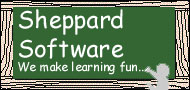Home | Software | Web Games | Quizzes | Free for Teachers | Patents | E-mail Us | Links
 Games Three and Four: Algebra Makes algebra fun!

Rules For Game Three: Algebra

You are given a formula, for example z = -y + 2. You are also given the values of x and y. From these values and the formula determine the correct anwer.

Example:
 x = 2 y = 1 z = ?
z = -y + 2

 ? = -6 -2 1 4 8

Solution:
 x = 2 y = 1 z = 1

z = -y + 2

Step 1. compute the value of ay: -1 times 1 = -1
Step 2. now add ay to b: -1 + 2 = 1

Click to play Game Three: Algebra

Rules For Game Four: Advanced Algebra

You must determine the correct function that is being used from the five functions listed. The correct function will apply to ALL of the numbers given in each column. For example, from the values of x and y below, how is the z value determined? Take a look:

 x = -7 -7 -1 0 2 2 6 9 y = -9 6 -2 1 0 3 5 -7 z = -7 6 -1 1 2 3 6 9
 z = Maximum(x,y) z = a|x| + b z = ax� + b z = axy+ b z = x�/y drop remainder

Solution: z = Maximum(x,y)

 x = -7 -7 -1 0 2 2 6 9 y = -9 6 -2 1 0 3 5 -7 z = -7 6 -1 1 2 3 6 9
 Step 1. determine the correct formula: z = Maximum(x,y) Step 2. -7 is the maximum (largest) of -7 and -9 Note: The formula z = Maximum(x,y) works for every one of the eight columns.

Click to play Game Four: Advanced Algebra